# Venn Diagram Examples for Problem Solving.Venn Diagram as a Truth Table

Create your Venn diagrams for logic problem solving using the ConceptDraw DIAGRAM diagramming and vector drawing software extended with the Venn Diagrams solution from the area "Diagrams" of ConceptDraw Solution Park.

"Venn diagram ... representing some sets by contours of closed shapes, such as circles or ellipses (and sometimes also the universal set as a rectangle enclosing all of these shapes), and indicating the relationships between the sets: by overlapping the shapes to show that the corresponding sets have a non-empty intersection, and by possibly (but not necessarily) enclosing all of the sets (which are proper subsets of the universal set) within a universal set (represented typically by a rectangle); such that the total number of simply connected regions is 2^n, where n is the number of depicted sets which are proper subsets of the universal set."
[Venn diagram. Wiktionary]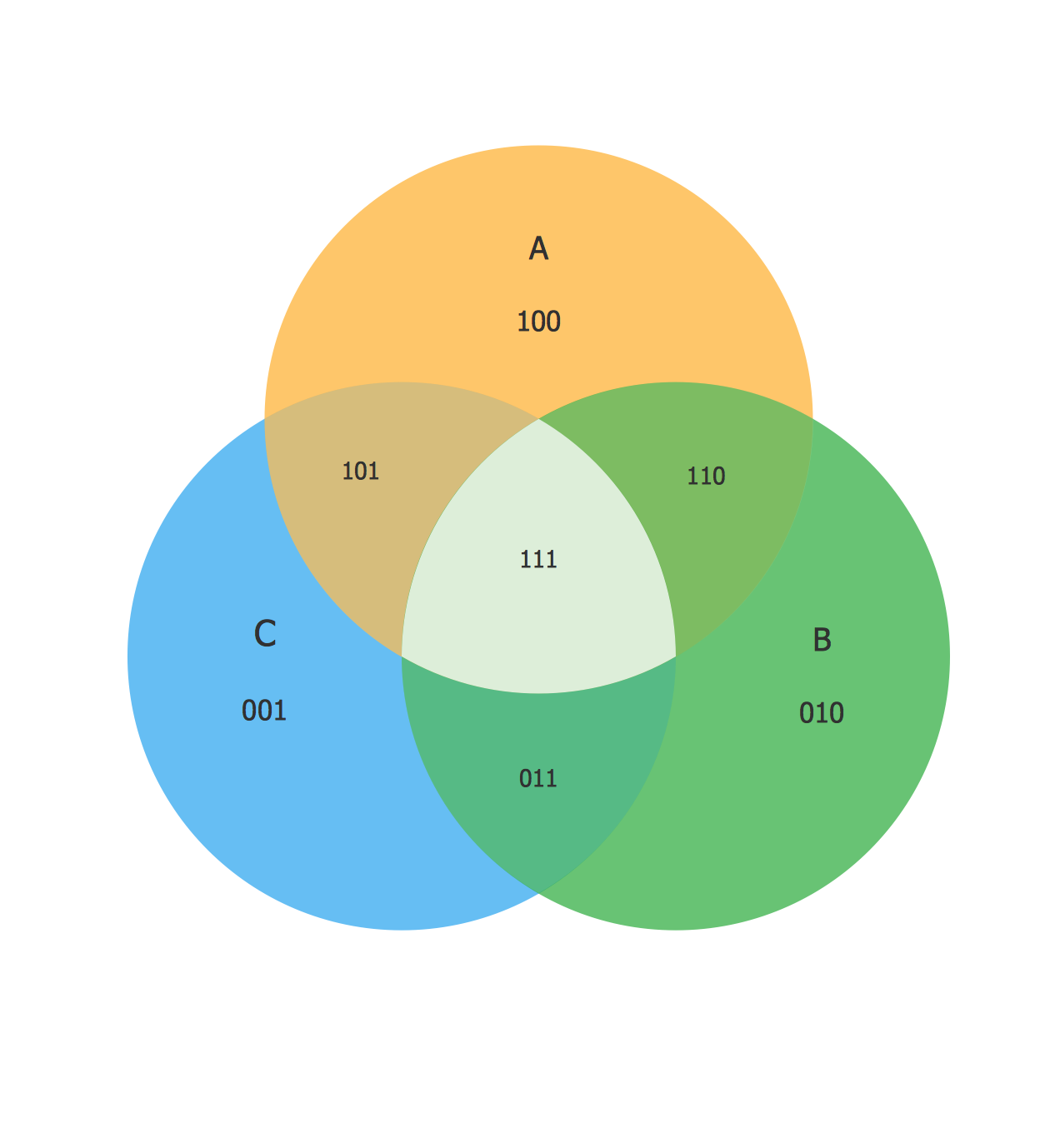Example 1. Venn diagram as a truth table.

"A truth table is a mathematical table used in logicâ€”specifically in connection with Boolean algebra, boolean functions, and propositional calculusâ€”to compute the functional values of logical expressions on each of their functional arguments, that is, on each combination of values taken by their logical variables (Enderton, 2001). In particular, truth tables can be used to tell whether a propositional expression is true for all legitimate input values, that is, logically valid."
[Truth table. Wikipedia]

The Venn diagram example above was redesigned from the Wikipedia file: Venn3tab.svg.
[en.wikipedia.org/wiki/File:Venn3tab.svg]

Related Solution:

### Program Evaluation and Review Technique (PERT) with ConceptDraw DIAGRAM v12

Big and complex projects sometimes need some simplification of plans and schedules. That's why Program Evaluation and Review Technique was invented and first implemented in 1958. You can create PERT diagrams effortlessly with ConceptDraw DIAGRAM v12 and share them with your colleagues. Program Evaluation Review Technique (PERT) is a method that is used to assess and analyze projects. PERT is a valuable tool for the project management practice . PERT gives an assessment and analysis of the time needed to the project completion. A PERT chart is a visual tool that delivers a graphical view of a project timeline. It is used to display the sequences and dependences of project tasks necessary to complete a project. ConceptDraw DIAGRAM v12 delivers the possibility to build a PERT along with other diagrams applied to assist management process by using its Seven Management and Planning Tools solution.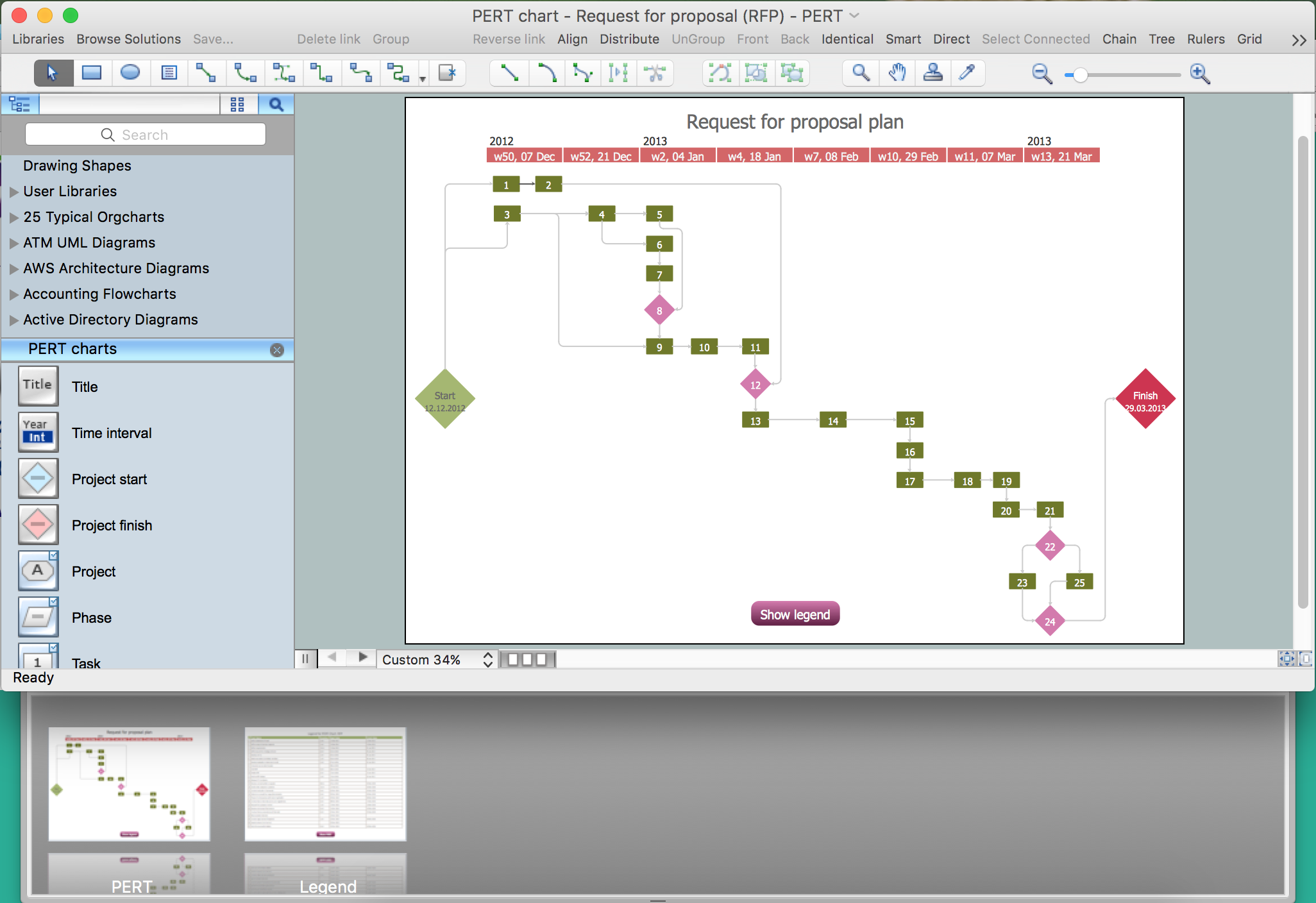Picture: Program Evaluation and Review Technique (PERT) with ConceptDraw DIAGRAM v12
Related Solution:

### 3 Circle Venn. Venn Diagram Example

This example shows the 3 Circle Venn Diagram. The Venn Diagrams visualize all possible logical intersections between several sets. On this example you can see the intersections of 3 sets. Venn Diagrams are widely used in mathematics, logic, statistics, marketing, sociology, etc.Picture: 3 Circle Venn. Venn Diagram Example
Related Solution:

### 2 Circle Venn Diagram. Venn Diagram Example

Using the ready-to-use predesigned objects, samples and templates from the Venn Diagrams Solution for ConceptDraw DIAGRAM v12 you can create your own professional looking Venn Diagrams quick and easy.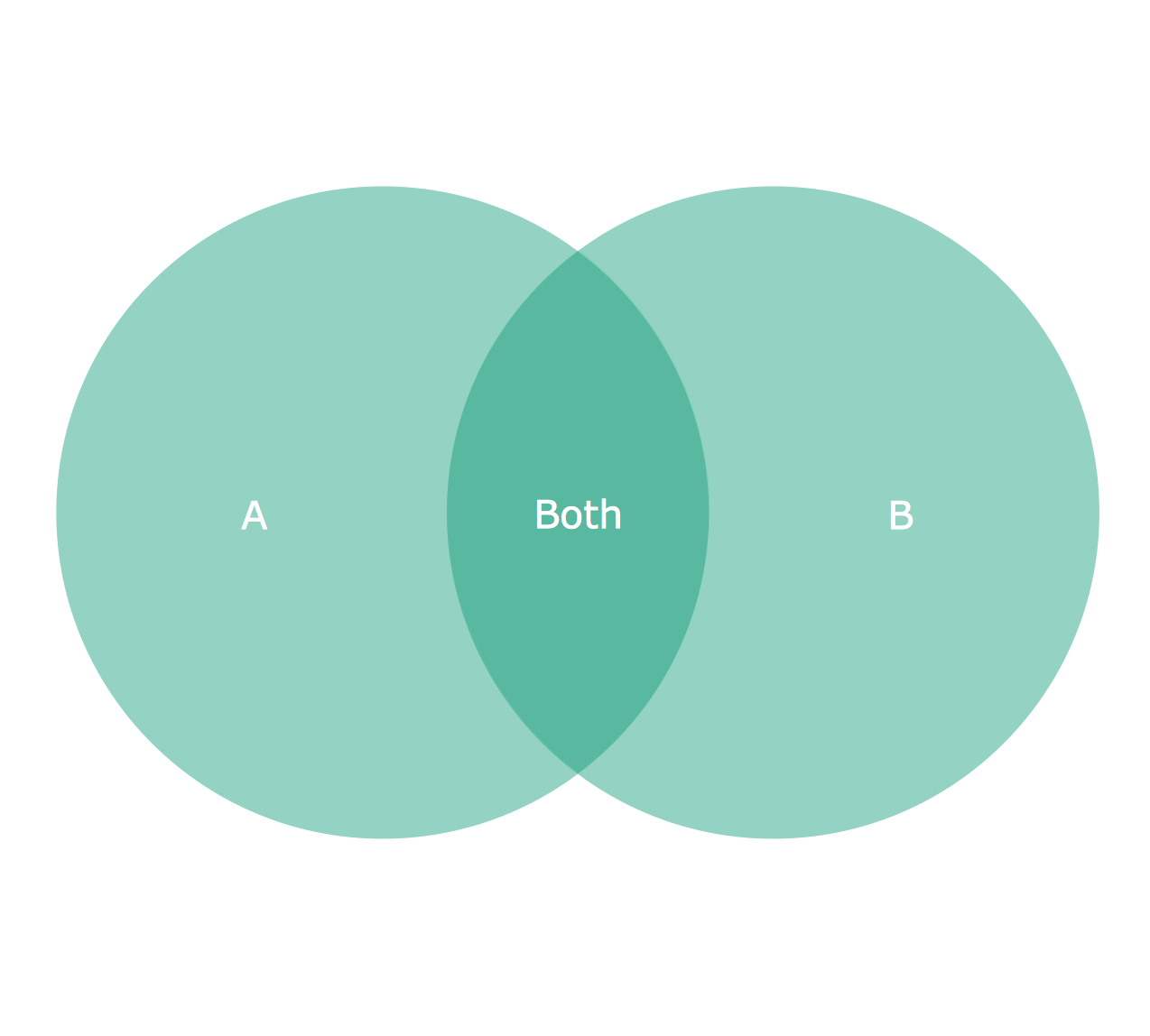Picture: 2 Circle Venn Diagram. Venn Diagram Example
Related Solution:

### UsingÂ Fishbone DiagramsÂ forÂ Problem Solving

Problems are obstacles and challenges that one should overcome to reach the goal. They are an inseparable part of any business, and the success of an enterprise often depends on ability to solve all problems effectively. The process of problem solving often uses rational approach, helping to find a suitable solution. Using Fishbone Diagrams for Problem Solving is a productive and illustrative tool to identify the most important factors causing the trouble. ConceptDraw DIAGRAM v12 extended with Fishbone Diagrams solution from the Management area of ConceptDraw Solution Park is a powerful tool for problem solving with Fishbone Ishikawa diagram graphic method.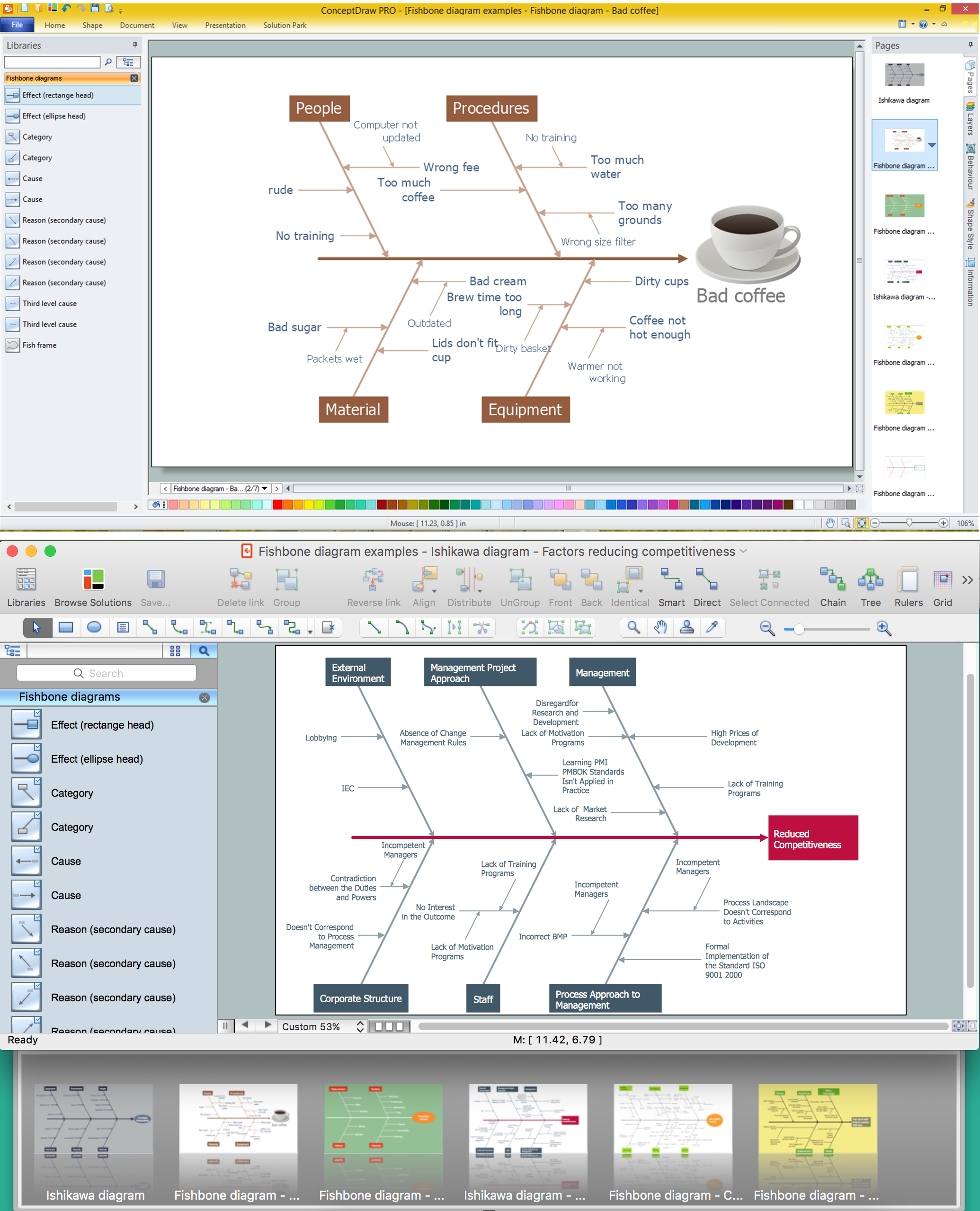Picture: UsingÂ Fishbone DiagramsÂ forÂ Problem Solving
Related Solution:

### Venn Diagram Template for Word

This template shows the Venn Diagram. It was created in ConceptDraw DIAGRAM v12 diagramming and vector drawing software using the ready-to-use objects from the Venn Diagrams Solution from the "What is a Diagram" area of ConceptDraw Solution Park.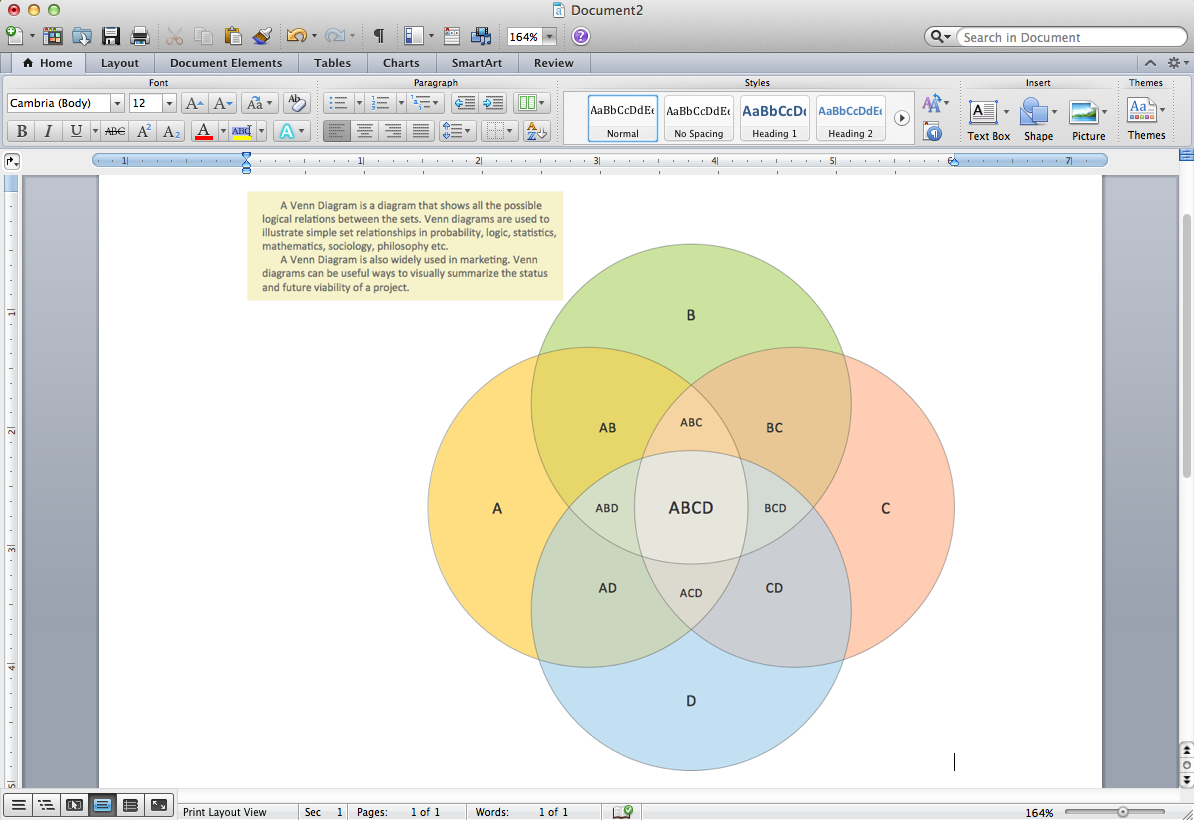Picture: Venn Diagram Template for Word
Related Solution:

### Cylinder Venn Diagram

You need design Cylinder Venn Diagram? Nothing could be easier with ConceptDraw DIAGRAM v12 diagramming and vector drawing software extended with Venn Diagrams Solution from the â€śWhat is a Diagramâ€ť Area. ConceptDraw DIAGRAM v12 allows you to design various Venn Diagrams including Cylinder Venn Diagrams.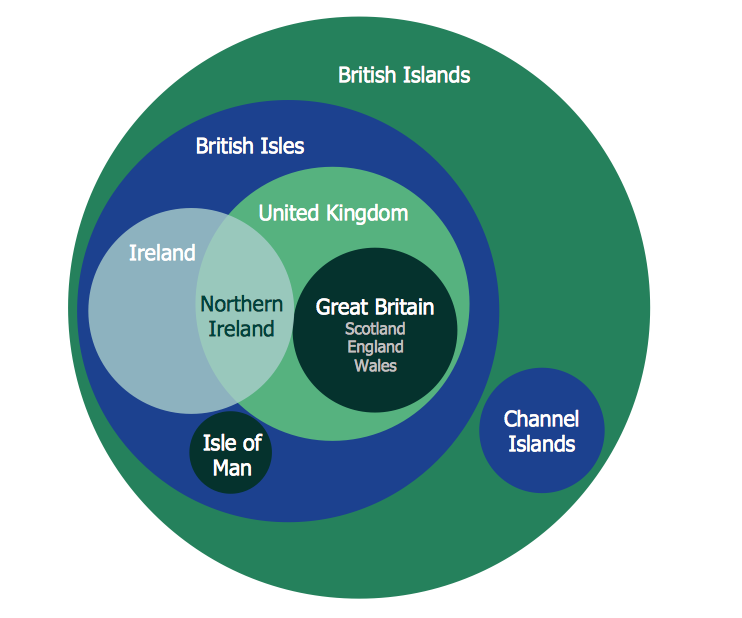Picture: Cylinder Venn Diagram
Related Solution:

### Venn Diagram Examples for Problem Solving. Computer Science. Chomsky Hierarchy

A Venn diagram, sometimes referred to as a set diagram, is a diagramming style used to show all the possible logical relations between a finite amount of sets. In mathematical terms, a set is a collection of distinct objects gathered together into a group, which can then itself be termed as a single object. Venn diagrams represent these objects on a page as circles or ellipses, and their placement in relation to each other describes the relationships between them. The Venn diagram example below visualizes the the class of language inclusions described by the Chomsky hierarchy.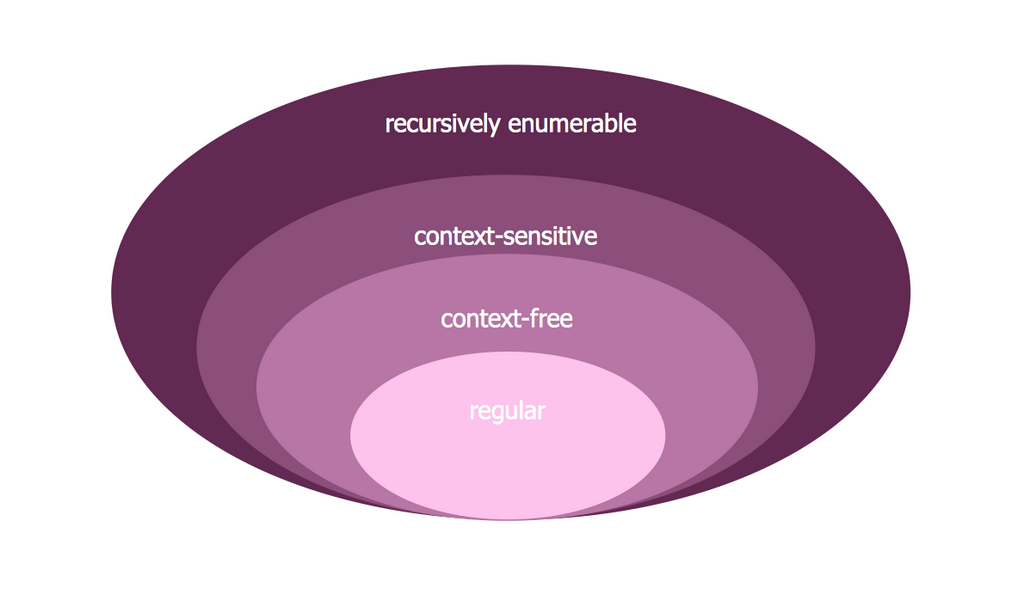Picture: Venn Diagram Examples for Problem Solving. Computer Science. Chomsky Hierarchy
Related Solution:

### Circuits and Logic Diagram Software

A circuit diagram is sometimes also called an elementary diagram, electronic schematic or electrical diagram circuits. It is essential in construction of any electronic equipment. Among many digital drawing tools available, ConceptDraw DIAGRAM v12 is a leading circuits and logic diagram software, affording for easy and quick creation of even the most advanced and complex diagram designs. This makes it a perfect choice for computer science and any kind of electronic engineering. This drawing includes the graphic symbols that may be in use while creating a logic circuit diagram. The diagrams of such kind are used in the electronics industry. The logic symbol depicts a device that realizes a Boolean type functions. Practically a logic symbol means transistor, diodes, relays, and other mechanical or optical details which provide function of closing or opening "gates". Totally the logic circuits can involve millions of gates. ConceptDraw Electrical Engineering solution gives the opportunity to create a circuit diagrams both simple and difficult.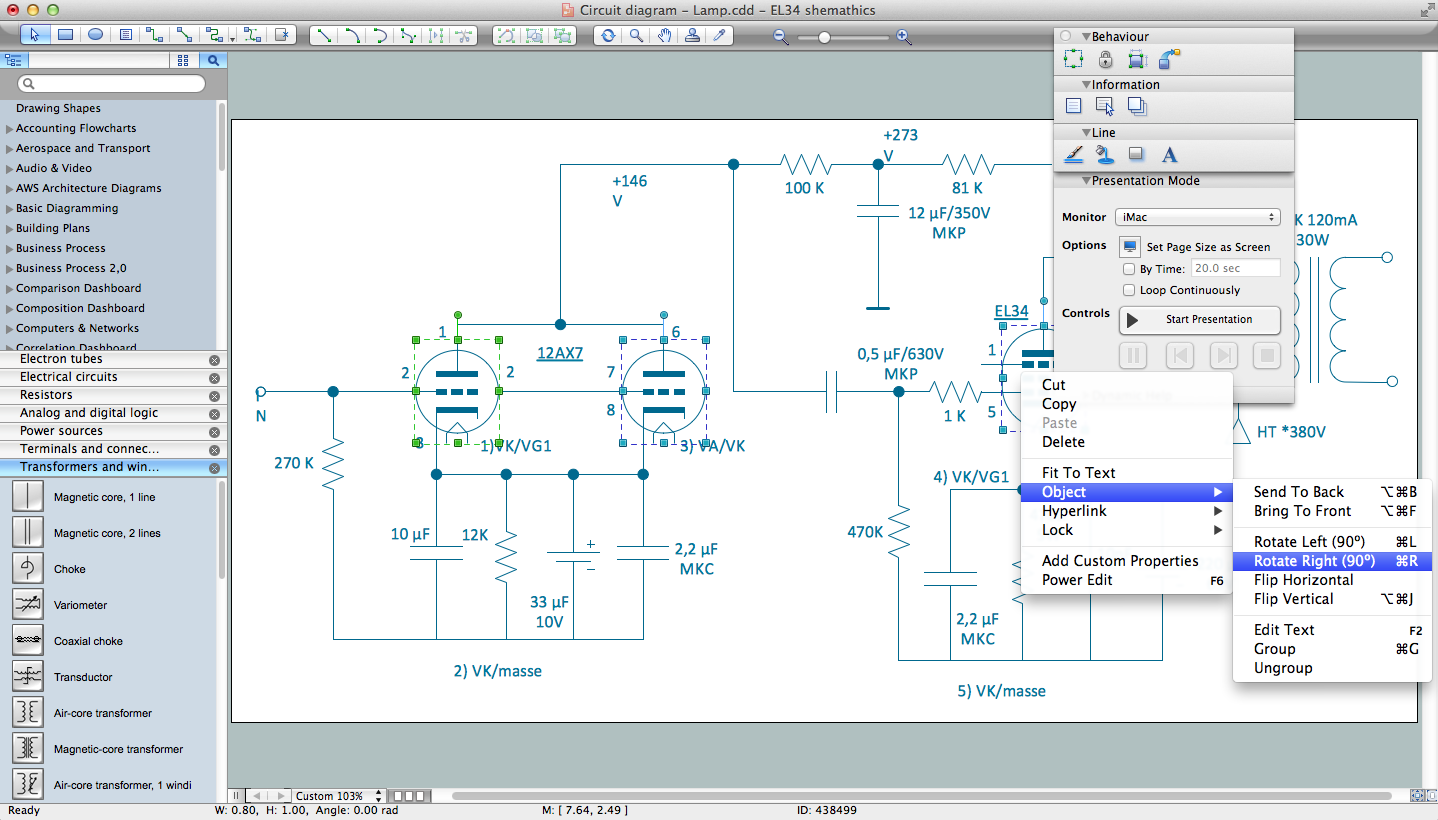Picture: Circuits and Logic Diagram Software
Related Solution:

### Venn Diagram

Venn diagrams are illustrations used in the branch of mathematics known as set theory. They show the mathematical or logical relationship between different groups of things (sets). A Venn diagram shows all the possible logical relations between the sets.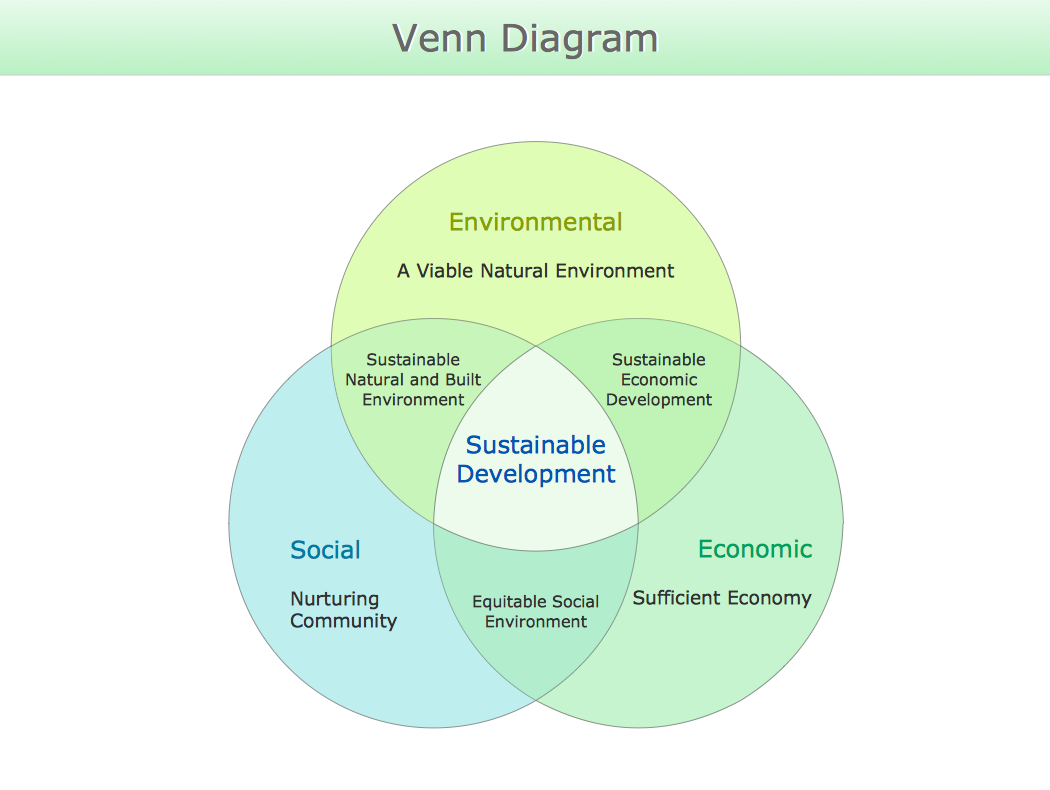Picture: Venn Diagram
Related Solution:

### 3 Circle Venn Diagram. Venn Diagram Example

This template shows the Venn Diagram. It was created in ConceptDraw DIAGRAM v12 diagramming and vector drawing software using the ready-to-use objects from the Venn Diagrams Solution from the "What is a Diagram" area of ConceptDraw Solution Park. Venn Diagrams visualize all possible logical intersections between several sets and are widely used in mathematics, logic, statistics, marketing, sociology, etc.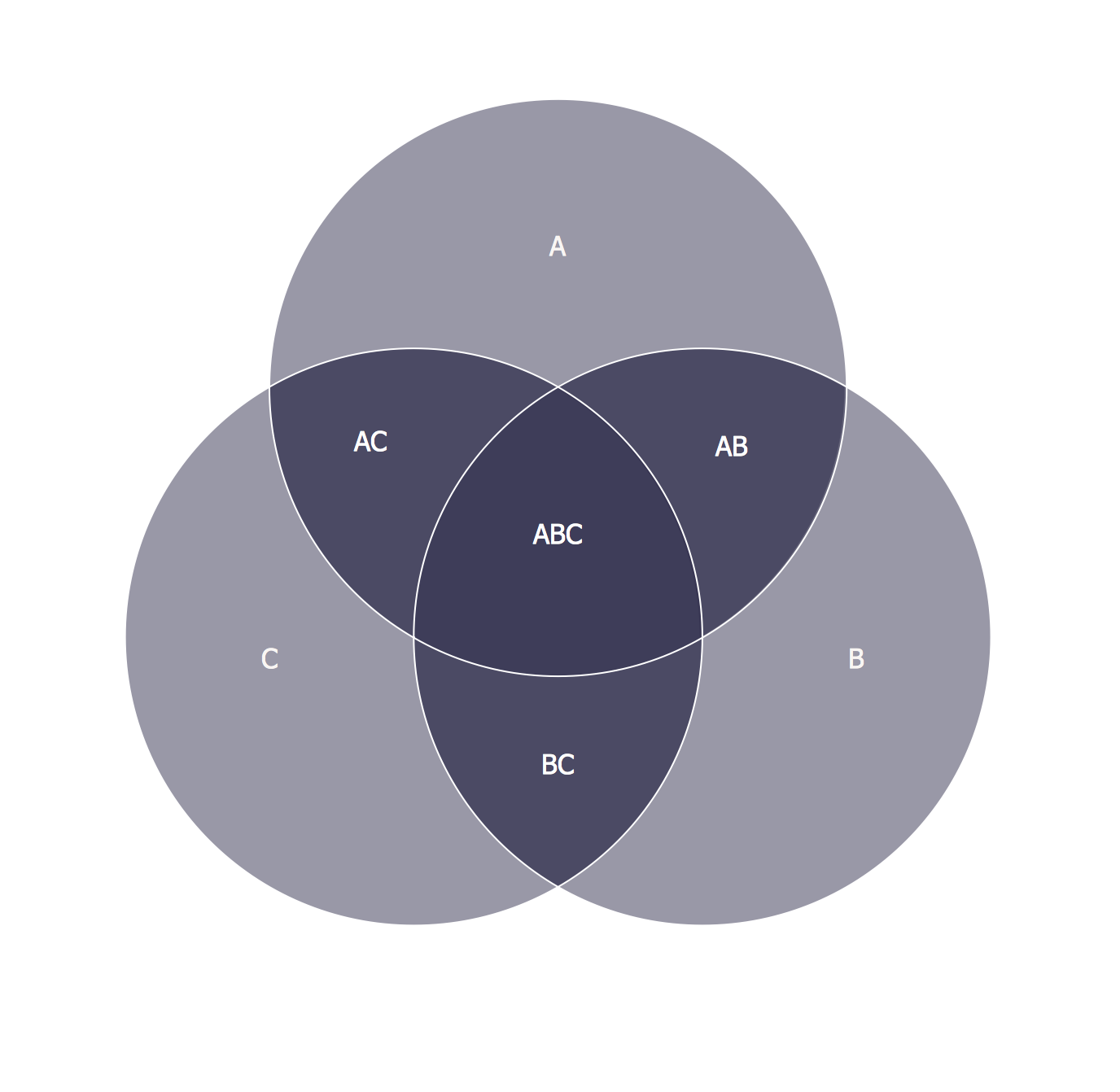Picture: 3 Circle Venn Diagram. Venn Diagram Example
Related Solution: